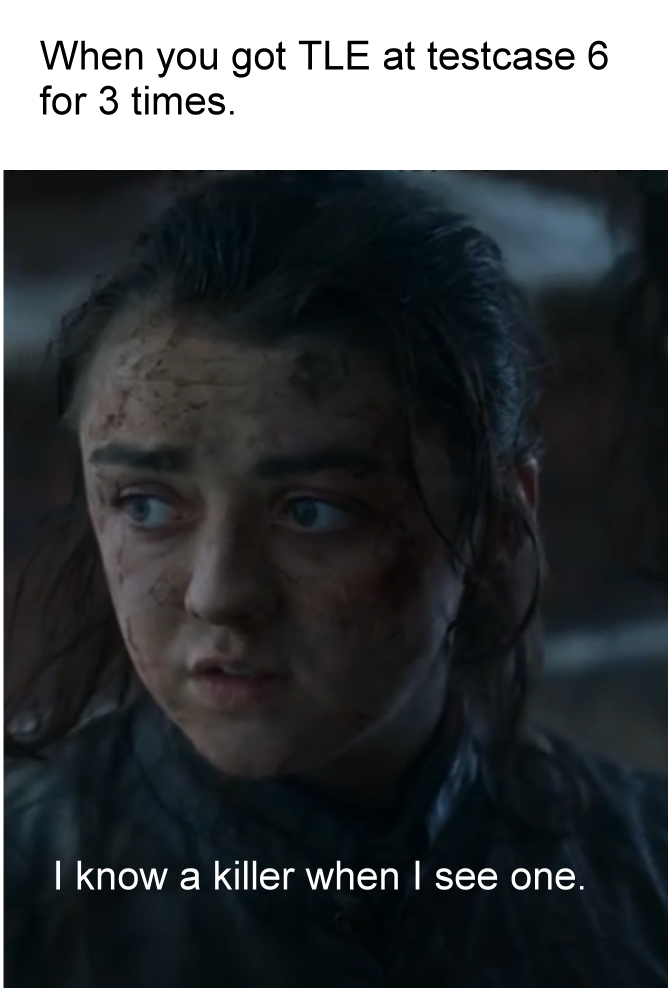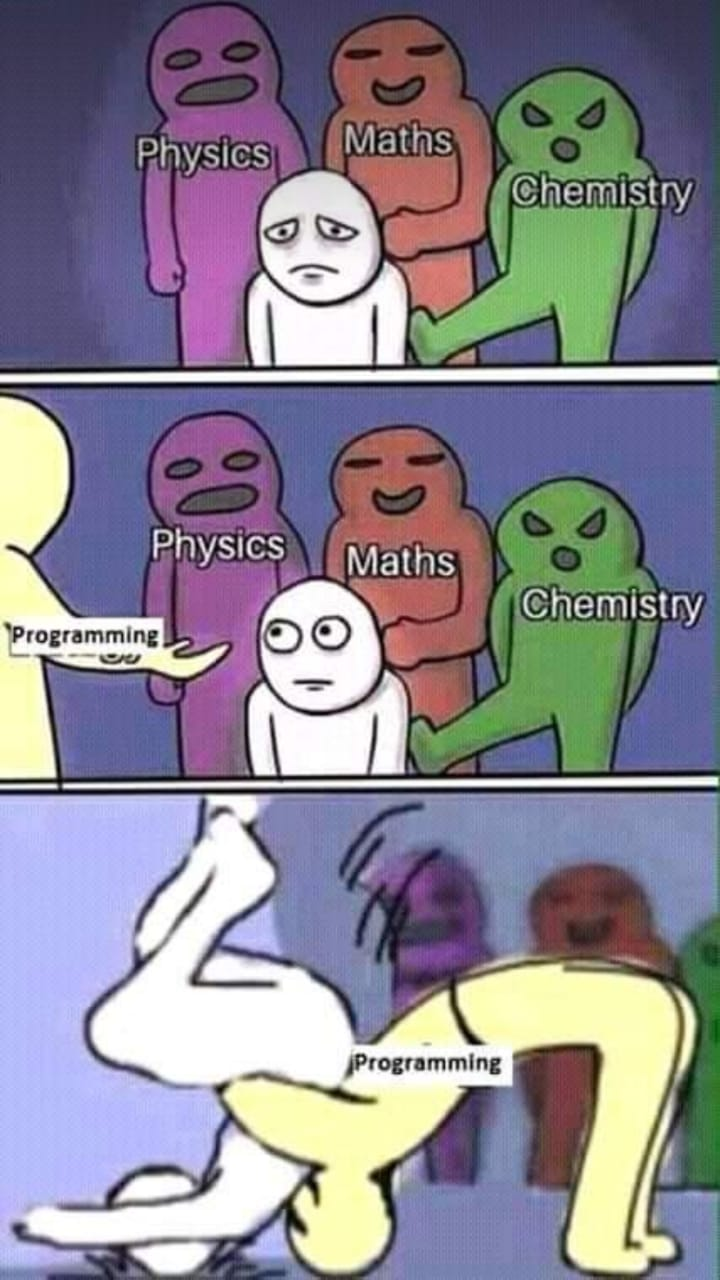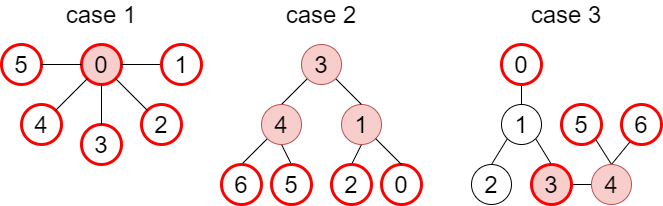# Time

 2021/06/22 18:40:00 2021/06/22 20:47:00

# Clarification

## 13225 - Guard The Wall - ver2

Status  |  Limits

### Description

I shall take no wife, hold no lands, father no children.

~ by anonymous loser.

The night's watch needs to guard The Wall.

But they're lack of resource. They can only choose q-3 men from q men to guard The Wall.

Help them find the maximum number of section they can protect.

There're n sections in a line numbered from 1 to n.

There're q soldiers, for i-th soldier, he can guard from section L_i to R_i(1 <= i <= q)

A section is guarded if at least one soldier guard the section.

You can only hire q-3 soldiers.

You need to find the maximum number of sections that can be guarded by hiring q-3 soldiers from q soldiers.

Example

If you got n = 4 sections and q = 4 soldiers.

First soldier guard L_1 = 1, R_1 = 1.

Second soldier guard L_2 = 2, R_2 = 2.

Third soldier guard L_3 = 3, R_3 = 4.

Forth soldier guard L_3 = 4, R_3 = 4.

You can hire q-3 = 1 soldier.

The best solution is hired the third soldier then you got 2 section guarded.### Input

The input first contains one integer t( 1 <= t <= 5) which means the number of testcases.

Each testcase first contains two numbers n, q(4 <= n, q <= 300).

Then the following q lines, each line contains two integer L_i, R_i( 1<= L_i <= R_i <= n )

### Output

For each testcase print a integer which means the maximum number of sections that can be guarded by hiring q-3 soldiers.

Remember to print \n at the end of each output.

## 13228 - Unlimited Triangle Work - ver2

Status  |  Limits

### Description

After obtaining enough swords, Gilgamesh would like to forge some more swords in his favorite form.

The requirements of forging a sword is to build a valid triangle: $x+y>z\text{ where }x, y, z\text{ is the sides}$. However, Gilgamesh prefers swords that forged by one more constrain: $x+y<1.5z$.

Now, he threats you with more swords that you forged, you have to help him.

You are given for positive integer $A\le B\le C\le D$, you're going to count how many eligible triangles can be build by edges with length $x, y, z$, where $A\le x\le B\le y\le C\le z\le D$.

A eligible triangle should follow the following condition:

1. $x+y>z$
2. $x+y<1.5z$

For example: $A=1, B=2, C=3, D=4$

You can build triangles with edges: $(x,y,z)\in\{(1,3,3),(2,2,3),(2,3,4)\}$

• Note that although $(2,3,3)$ is a valid triangle, it does not fit the 2-nd constrain: $x+y<1.5z$ ($2+3=5>1.5\times 3=4.5$).

### Input

The first line contains one integer $T$, there are $T$ testcases below.

For each testcase, the four integer $A,B,C,D$ is given respectively.

$1\le T\le 100$.

$1\le A\le B\le C\le D\le 5\times 10^4$.

### Output

For each testcase, output its answer, followed by a newline character.

## 13229 - Never gonna give you up

Status  |  Limits

### Description

Never gonna give you up~

Never gonna let you down~

Never gonna run around and desert you~

~ by Rick Astley

Rick will never let you down, but today he will let you count.

He's no stranger to count.

You know the rules and so you code.

A full commitment of coding is what you are thinking of.

You wouldn't get this from any other guy.

You just wanna tell Rick how you're coding.

Gotta make Rick understand.

(video support)

You got n numbers ai( 1 i n) and a number k denotes a number system with base k.

You are ask to figure out how many different last digit can be found by the

sum of several ai (Σaiwi for wi∈{N,0},1≤i≤n) (p.s. N means natural number )

in the number system with base k.

Output how many number of digits you can found and output all the digits in increasing order.

Example

If you got n = 2 and k = 8 .

{ ai | 1 ≤ i ≤ 2 }= { 12, 20 }

12 can be written as 14 in the number system with base 8, thus we found 4.

20 can be written as 24 in the number system with base 8 , thus we found 4.

20 + 12 = 32 can be written as 40 in the number system with base 8 , thus we found 0.

20 + 20 + 12 = 52 can be written as 64 in the number system with base 8 , thus we found 4.

........

After trying all possible number of 20w1 + 12w(w1, w ∈{N,0} ) we found that

we can only found {0, 4}.

You then need to output the answer:

2

0 4

Hint

If you try to brute force list all the possible number of linear combination of ai you won't solve this problem!### Input

The input will end by EOF.

There will be multiple queries in one testcase but for each testcase there won't exceed 10 queries.

For each queries, there are two lines.

First line contains two integer n, k (1 n 105, 2 k 105).

Second line contains n integer ai ( 1 ai 109 ).

### Output

For each queries the output contains two lines.

The first line contains the number of different last digit can be found.

The second line contains the different last digit you found.

Seperate each number by one blank, note that there's no blank follow the last number.

Remember to print \n at the end of each line.

## 13242 - Poorgrammer - ver2

Status  |  Limits

### Description

A program a day keep girls away.

~by anonymous programmer

You are a programmer, you need to write code day and night.

In order to keep you in a sharp mind, you drink a lot of coffee.

Given n cups of coffee, the ith cup of coffee has the effect that make

you write ai lines of code.

Each cup of coffee can only be drunk for once.

You can drink coffee in arbitary order!!!!

You need to write more than m lines of code.

In a day when you drink a cup of coffee,

the second cup of coffee will loss it's effect by 1

and the third cup of coffee will loss it's effect by 2

..... and so on

You need to find out what's the minimum number of days

that you can write more than m lines of code.

Example:

Given n = 5 cups of coffee, you need to finish m=16 lines of code.

The effect of coffee = a1 = 5, a2 = 5, a3 = 5, a4 = 5, a5 = 5

If you drink a1~a4 at the first day, you finish 5 + (5-1) + (5-2) + (5-3) = 14 lines of code.

If you drink a5 at the second day, you finish 5 lines of code.

14+5 > 16 therefore you can finish your code by 2 days,

and it's the minimum number of days that you can achieve.### Input

First line contains one integer t(1 <= t <= 10) which means the number of testcases.

Each testcases contains two lines.

First line contains two integer n(1 <= n <= 2*10^5), m(1 <= m <= 10^9)

Second line contains n numbers ai(1 <= ai <= 10^9)

### Output

For each testcase print only one number which means the minimum number of days

that you can write more than m lines of code.

If you can't finish your code in any way, print -1.

Remember to print \n at the end of output.

## 13243 - AI Composer

Status  |  Limits

### Description

I want my singing to bring happiness to everyone.

── Vivy

Vivy is a songstress AI, who put on a performance at the amusement park NiaLand in front of a small audience. Her duty is to make every happy with my singing. After she meets a cube AI, Matsumoto, who came from 100 years into the future, her centennial journey begins...

However, she's sill a songstress AI, whatever Matsumoto asks her to do, her duty never change: to make every happy with her singing. Therefore, she wants to create her own song.

After a lot of effort, she finally compose the melody of her song, but she doesn't have any idea about the lyrics. You are a copy-paste AI, and you're asked to help her fill the lyrics in.

You've searched a bunch of lyrics along with its melody. What you need to do is to copy the lyrics to the corresponding melody that Vivy creates. Can you help her?

You will be given two strings $s$ and $t$, the length of which is $n$ and $m$, respectively. The strings contain only lowercase letters (i.e. a-z).

You can copy any number of any substring of $s$, and you can reverse the substrings. Your task is to reconstruct string $t$ with the copied substrings of $s$.

• A substring of a string is a consecutive segmentation of a string. For example, cde is a substring of bcdefg, bde is not. And we call cde substring $[2,4]$ of string bcdefg.
• The reversed substring is a substring that been reversed. For example, edc is a reversed substring of bcdefg. And we call edc reversed substring $[4,2]$ of string bcdefg.

For simplicity, we want the number of substrings as small as possible.

For example, the first case:

3
xyz
6
xyzzyx


We can slice a substring $[1,3]$ of $s$ (xyz) and a reversed substring $[3,1]$ of $s$ (zyx) and reconstruct the desired string $t$, so the minimum number of substrings is $2$.

Note that although we can slice $[1,2]$, $[3,3]$, and $[3,1]$ to get the string $t$, the number of substrings is not minimum.

### Input

The first line is an integer $T$, indicates $T$ testcases below.

For each testcase, there are $n,\ s,\ m,\ t$ line by line, respectively.

$1\le T\le 20$.

$n=|s|,\ m=|t|,\ 1\le n,m\le 3000$.

### Output

For the first line of each testcase, output the minimum number of substrings.

For the next several lines, each line output the interval $l$ and $r$ of substrings, separated by space.

• $l$ and $r$ are the left and right boundaries of a substring. For usual substrings, output $l\le r$, and $l\ge r$ for reversed substrings. Please refer to sample input and output.

If there are multiple answers, you may output any of them. For example, both slices $[3,2]$, $[9,8]$, $[3,6]$, $[10,10]$ and slices $[3,1]$, $[8,8]$, $[3,6]$, $[10,10]$ are acceptable for the second testcase, you can output any of them.

## 13245 - I2P(I) 2021_Chen_final_rule

Status  |  Limits

### Description

1. Only C only allowed. Solving problems with other languages (e.g. python, java) are forbidden, otherwise you'll get zero point.

2. Paper references, electronic devices, or other items that may contain any information relative to this exam are not allowed.

3. Before leaving, please tell TAs, we'll check if your accepted / partially accepted submissions are all written in C . After you pass the check, you may sign your name as a record and leave.

4. The score of each problem is shown below (final exam / final HW):

• 13225: 9 points / 7 points

• 13228: 9 points / 7 points

• 13229: 7 points / 4 points

• 13242: 9 points / 7 points

• 13243: 4 points / 3 points

• 13246: 5 points / 2 points

If you get partially correct in a problem, your score of the problem will be

• score of the problem * number of testcases you passed / number of testcases

For example, if score of a problem is 10 points and the problem contains 6 testcases. If you pass the 3 testcases, you'll get 10*3/6=5 points on this problem.

## 13246 - Youbike Racing Tournaments

Status  |  Limits

### Description

The world's largest youbike racing contest is holding...... again! It's time to learn to youbike again...

However, the judgements and organizers decide to change the rule of competition for the second season: intercity youbike racing contest - youbike racing tournaments!

The youbikers are divided into groups by their cities, each group stays in its own city. If two groups are going to compete, both of the groups have to move to the assigned venue city and compete each other.

For the first round, the organizers decide to hold a knockout stage. That is, two groups compete each other, and the lose one is eliminated.

You are one of the organizers, who is assigned to make decision of the complete groups and location of venue cities. Note that if the venue city of two knockout competition is held in the same city, the organizers will cost less, so the host wants the number of venue cities as small as possible.

Given a map with $n$ nodes, $n-1$ edges, and $2m$ special nodes. You need to:

1. Divide special nodes into $m$ groups, for number of each is 2.
2. For each group, pick one node that lies in the path of the two nodes of the group. The picked node can also choose the start point and end point of the path.
3. The picked node of different groups can be the same.
4. We want the number of picked nodes as small as possible.

We grantee that:

1. There's only and exactly one path from any node $A$ to any other node $B$.

For example:The nodes with bold red frame are the special nodes, and the nodes filled with red are possible choices of picked node.

The minimum number of picked nodes are 1 for the three cases. For case 2, we can pick node 3 as our picked node, where node 3 lies in the path of (6,2) and (5,0), and so do node 4 and 1.

### Input

The first line contains an integer $T$, there are $T$ testcases below.

For each testcase:

1. The first line contains two integers $n$ and $m$.
2. Then $n-1$ lines below, each line contains two integers $a$ and $b$, indicates there's a path from node $a$ to node $b$ (and node $b$ to node $a$).
3. The last line contains $2m$ integers, indicate the special nodes.

$1\le T\le 20$, $2\le n\le 3000$, $2\le 2m\le n$.

### Output

For each testcase, output:

1. First line contains the total number of picked nodes.
2. Second line output the indices of the picked nodes.
3. Then $m$ lines below. Each line contains three integers, which is two special nodes that divided to the same group and their picked node respectively, separated by a space.

If there are multiple answers (i.e. same total number of picked nodes but with different node indices), you may output any of them.